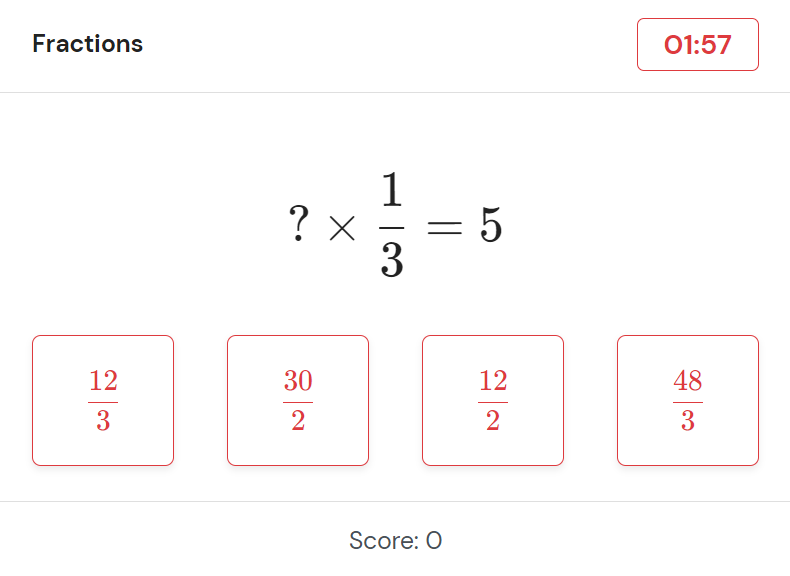# Improve your mental math, fast.

Practice representative mental math problems in an environment that mimics real trading interviews. Join thousands of applicants in ensuring the best and most efficient preparation for your numerical test.Become a trader by practicing your mental math in an environment that mimics real trader interviews.

### Progress Tracking

Monitor your progression and find your weak spots by tracking your results over time.

### Targeted Practice

Strengthen your weak points by focusing on specific question types.

### Integers

In order to ace your trading interview it is essential to get the basics right. We offer standard arithmetic operations with integers and adjust the question and answer type depending on the company that you want to prepare for.

Examples
• $$-66 + 105 =$$
• $$5 \times 18 =$$
• $$109 - 46 =$$
• $$170 \medspace/\medspace 5 =$$

### Decimals

Mastery of arithmetic operations with decimals is vital for aspiring traders. Our practice sets include precisely those variations that are relevant for trading interviews.

Examples
• $$0.16 + 23.01 =$$
• $$2.5 \times 0.09 =$$
• $$-6.63 - 3.593 =$$
• $$21 \medspace/\medspace 0.7 =$$

### Large multiplications

Flow Traders is known to emphasize large multiplications in a multiple choice format. Our Red Test therefore includes such questions in order to familiarize aspiring traders with them.

Example
• $$11011 \times 11011 =$$
• $$800 \times 8000 =$$
• $$0.16 \times 23812 =$$
• $$42.5 \times 1.04 =$$

### Fractions

The '80 in 8' test employed by Optiver includes various types of computations with fractions. Our Orange Test allows you to familiarize yourself with such questions and save you valuable seconds during your numerical test.

Examples
• $$\frac{4}{9} + \frac{10}{27} = \text?$$
• $$\text? \times \frac{1}{8} = 2$$
• $$\frac{3}{9} - \text? = \frac{5}{18}$$
• $$\frac{2}{20} \medspace/\medspace \frac{1}{5} = \text?$$

## Practice Mode

Use our Practice Mode to improve your performance on specific types of questions. Push yourself to correctly answer as many questions as possible within 2 minutes.## Test Mode

Make your numerical test feel like routine work by practicing in Test Mode. Our Test Mode is designed to mimic the appearance, scoring, and types of questions employed by real trading firms. Choose our Red Test when preparing for the numerical test of Flow Traders. If you have Optiver's '80 in 8' coming up, go for our Orange Test instead. Please note that we are not in any way affiliated with either of these companies.## Our subscriptions

Choose between two weeks or a full month access.

Subscribers enjoy unlimited tests, targeted practice and progress tracking.

### \$34.95